## Workshop on Bootstrapping and Bayesian Econometrics

The goal of this workshop is to 1. Explore bootstrapping * See the implementation steps * Show subtleties of reported results 2. Introduce Bayesian Econometrics * Brief discussion of Bayes Rule * Markov Chains and the Metropolis-Hastings Sampler * Simple example estimating mean and standard deviation * Application to Tobias and Koop * Heirarchical Models * Show you three different samplers

In :
#load python libraries for this ipython notebook:
import numpy as np              # numpy linear algebra library
import pandas as pd             # pandas data manipulation library
import emcee as emcee           # a bayesian library
#load pymc3 libraries (another Bayesian Library)
import imp


# Estimation methods in Econometrics

In class, we looked at these estimation methods: 1. Linear Model 2. Maximum Likelihood 3. Generalized Method of Moments

Leaving these as major estimation paradigms you haven't seen: 4. Bayesian Estimation 5. Non-parametric Estimation

In what follows, we will replicate our Bootstrapping work in Ipython (so we can compare to Bayesian Methods) and then move on to Bayesian Estimation.

Note: the appeal of Bayesian modeling is to tackle computationally challenging models and the implementation of an OLS model is fairly trivial, but gives us a point of comparison since we know the OLS model very well. At the end of these notes, we show how Bayesian Econometrics opens the door to very rich models, that are difficult to replicate using frequentist methods.

## Load Tobias and Koop data for time==4

In :
tobias_koop=pd.read_csv('http://rlhick.people.wm.edu/econ407/data/tobias_koop_t_4.csv')

In :
tobias_koop.head()

Out:
id educ ln_wage pexp time ability meduc feduc broken_home siblings pexp2
0 4 12 2.14 2 4 0.26 12 10 1 4 4
1 6 15 1.91 4 4 0.44 12 16 0 2 16
2 8 13 2.32 8 4 0.51 12 15 1 2 64
3 11 14 1.64 1 4 1.82 16 17 1 2 1
4 12 13 2.16 6 4 -1.30 13 12 0 5 36
In :
tobias_koop.describe()

Out:
id educ ln_wage pexp time ability meduc feduc broken_home siblings pexp2
count 1034.000000 1034.000000 1034.000000 1034.000000 1034 1034.000000 1034.000000 1034.000000 1034.000000 1034.000000 1034.000000
mean 1090.951644 12.274662 2.138259 4.815280 4 0.016596 11.403288 11.585106 0.169246 3.200193 27.979691
std 634.891728 1.566838 0.466280 2.190298 0 0.920963 3.027277 3.735833 0.375150 2.126575 22.598790
min 4.000000 9.000000 0.420000 0.000000 4 -3.140000 0.000000 0.000000 0.000000 0.000000 0.000000
25% 537.250000 12.000000 1.820000 3.000000 4 -0.550000 11.000000 10.000000 0.000000 2.000000 9.000000
50% 1081.500000 12.000000 2.120000 5.000000 4 0.170000 12.000000 12.000000 0.000000 3.000000 25.000000
75% 1666.500000 13.000000 2.450000 6.000000 4 0.720000 12.000000 14.000000 0.000000 4.000000 36.000000
max 2177.000000 19.000000 3.590000 12.000000 4 1.890000 20.000000 20.000000 1.000000 15.000000 144.000000
In :
# explore distribution of ln_wage (dv)
tobias_koop.ln_wage.plot(kind='hist')

Out:
<matplotlib.axes.AxesSubplot at 0x51b7dd0>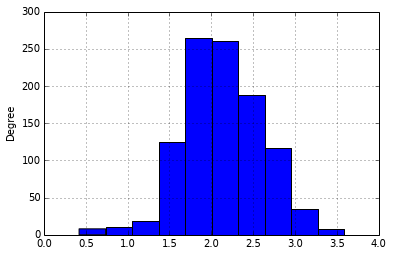In :
# run OLS regression:
results = pd.ols(y=tobias_koop['ln_wage'], x=tobias_koop[['educ','pexp','pexp2','broken_home']])
results

Out:

-------------------------Summary of Regression Analysis-------------------------

Formula: Y ~ <educ> + <pexp> + <pexp2> + <broken_home> + <intercept>

Number of Observations:         1034
Number of Degrees of Freedom:   5

R-squared:         0.1664

Rmse:              0.4265

F-stat (4, 1029):    51.3606, p-value:     0.0000

Degrees of Freedom: model 4, resid 1029

-----------------------Summary of Estimated Coefficients------------------------
Variable       Coef    Std Err     t-stat    p-value    CI 2.5%   CI 97.5%
--------------------------------------------------------------------------------
educ     0.0853     0.0093       9.18     0.0000     0.0671     0.1035
pexp     0.2035     0.0236       8.63     0.0000     0.1573     0.2497
pexp2    -0.0124     0.0023      -5.44     0.0000    -0.0169    -0.0079
broken_home    -0.0087     0.0357      -0.24     0.8070    -0.0787     0.0613
intercept     0.4603     0.1373       3.35     0.0008     0.1912     0.7294
---------------------------------End of Summary---------------------------------


These s.e.'s aren't robust (don't match stata output), so let's bootstrap. The statsmodels package has a robust and bootstrap option (see http://statsmodels.sourceforge.net/stable/rlm.html for the robust OLS model). In general, you should use the canned routines, but we present the following for educational purposes only to make explicit what the bootstrap is doing.

Steps: 1. Loop $R$ times ($R$ = # of boostrapped replicates) 2. For each $r\in R$, sample $N$ times with replacement from $\mathbf{Y}$ and $\mathbf{X}$, noting that $N$ is equal to the number of observations in the original dataset $\mathbf{Y}$ and $\mathbf{X}$. Denote each of these samples as $\mathbf{X}_r$ and $\mathbf{Y}_r$ 3. Estimate an OLS model and recover estimate for replicate $r$: $\hat{\beta}_r = (\mathbf{X}_r'\mathbf{X}_r)^{-1}\mathbf{X}_r'\mathbf{Y}_r$ 4. Store results 5. Calculate the standard deviation (our estimate for the s.e.) of each parameter estimate, or construct non-parametric confidence intervals (preferred)

In :
R = 400

results_boot = np.zeros((R,results.beta.shape))

row_id = range(0,tobias_koop.shape)

for r in range(R):
this_sample = numpy.random.choice(row_id, size=tobias_koop.shape, replace=True) # gives sampled row numbers
# Define data for this replicate:
tobias_koop_r = tobias_koop.iloc[this_sample]
# Estimate model
results_r = pd.ols(y=tobias_koop_r['ln_wage'], x=tobias_koop_r[['educ','pexp','pexp2','broken_home']])
# Store in row r of results_boot:
results_boot[r,:] = np.asarray(results_r.beta)

In :
# Convert results to pandas dataframe for easier analysis:
results_boot = pd.DataFrame(results_boot,columns=['b_educ','b_pexp','pexp2','b_broken_home','b_Intercept'])

In :
results_boot.head(10) # the first 10 replicates

Out:
b_educ b_pexp pexp2 b_broken_home b_Intercept
0 0.086845 0.209767 -0.013187 0.042530 0.424621
1 0.055819 0.151736 -0.007761 -0.058262 0.931521
2 0.075879 0.137397 -0.006732 -0.010441 0.719790
3 0.073911 0.205536 -0.013938 -0.106334 0.662189
4 0.082670 0.209667 -0.012387 -0.071467 0.430717
5 0.079207 0.218031 -0.014434 0.026968 0.548299
6 0.110818 0.183666 -0.009843 0.012264 0.156372
7 0.098079 0.211655 -0.013272 0.005513 0.276762
8 0.060890 0.220493 -0.016071 -0.115970 0.799638
9 0.102324 0.198275 -0.011950 0.064837 0.212105
In :
results_boot.b_educ.plot(kind='hist')

Out:
<matplotlib.axes.AxesSubplot at 0x533ced0>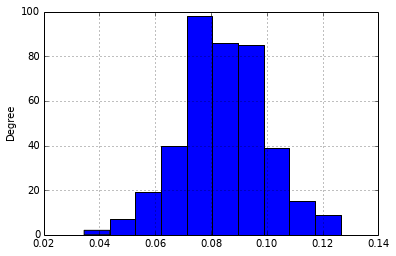In :
results_boot.describe(percentiles=[.005,.025,.05,.5,.95,.975,.995])

Out:
b_educ b_pexp pexp2 b_broken_home b_Intercept
count 400.000000 400.000000 400.000000 400.000000 400.000000
mean 0.084648 0.205918 -0.012596 -0.014049 0.462193
std 0.015267 0.036862 0.003708 0.051261 0.209663
min 0.034552 0.045842 -0.023124 -0.156739 -0.213324
0.5% 0.044082 0.099568 -0.021045 -0.134710 -0.071113
2.5% 0.053761 0.129801 -0.019293 -0.117726 0.051967
5% 0.059072 0.145110 -0.018207 -0.096306 0.111766
50% 0.084729 0.207228 -0.012590 -0.014617 0.454001
95% 0.110858 0.262188 -0.006414 0.073874 0.824146
97.5% 0.115970 0.276534 -0.004944 0.094848 0.891660
99.5% 0.123583 0.295535 -0.001668 0.126296 0.962410
max 0.126530 0.308454 0.003527 0.132844 1.098337

Let's plot our bootstrap replicates, by parameter. Note that the values of each of these replicates are completely independent (or should be). Replicate 3 doesn't in any way inform us about replicate 4.

In :
replicate= np.arange(R)

# plot point estimate and confidence intervals:
plt.figure(figsize=(14, 10), dpi=200)
lw = 1

plt.subplot(521)
plt.ylabel("Intercept")
plt.plot(replicate, results_boot.b_Intercept, label="Intercept",lw=lw)
plt.subplot(522)
plt.hist(results_boot.b_Intercept,lw=lw, label="Intercept", orientation='horizontal')

plt.subplot(523)
plt.ylabel("b_educ")
plt.plot(replicate, results_boot.b_educ, label="b_educ",lw=lw)
plt.subplot(524)
plt.hist(results_boot.b_educ,lw=lw, label="b_educ",orientation='horizontal')

plt.subplot(525)
plt.ylabel("b_pexp")
plt.plot(replicate, results_boot.b_pexp, label="b_pexp",lw=lw)
plt.subplot(526)
plt.hist(results_boot.b_pexp,lw=lw, label="b_pexp",orientation='horizontal')

plt.subplot(527)
plt.ylabel("b_pexp2")
plt.plot(replicate, results_boot.b_pexp, label="b_pexp2",lw=lw)
plt.subplot(528)
plt.hist(results_boot.b_pexp,lw=lw, label="b_pexp2",orientation='horizontal')

plt.subplot(529)
plt.ylabel("b_broken_home")
plt.plot(replicate, results_boot.b_broken_home, label="b_broken_home",lw=lw)
plt.subplot(520)
plt.hist(results_boot.b_broken_home,lw=lw, label="b_broken_home",orientation='horizontal')

Out:
(array([  5.,  13.,  35.,  67.,  84.,  96.,  53.,  28.,  12.,   7.]),
array([-0.15673906, -0.12778079, -0.09882252, -0.06986425, -0.04090598,
-0.01194771,  0.01701055,  0.04596882,  0.07492709,  0.10388536,
0.13284363]),
<a list of 10 Patch objects>)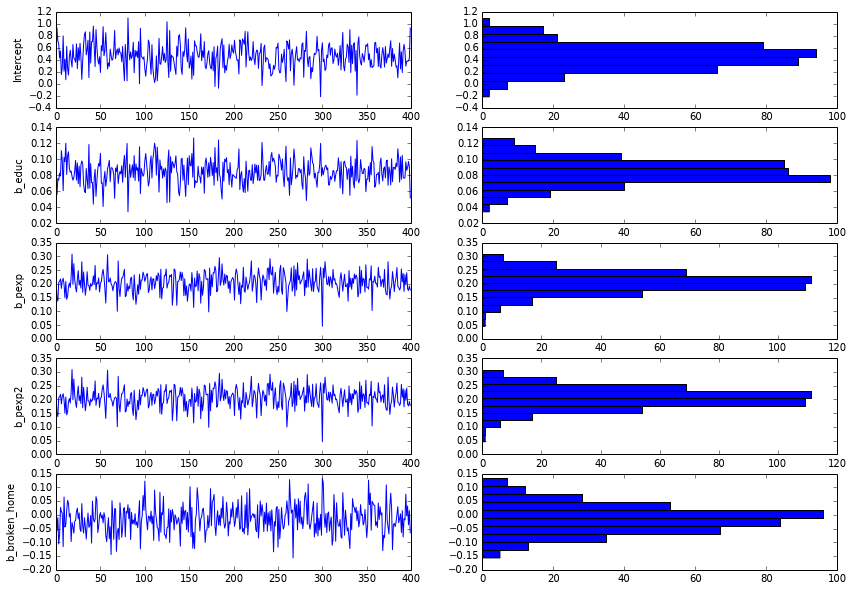# Now for Bayesian Estimation of the same model:

The Bayesian approach is functionally similar yet comes at the problem from a philosphically different viewpoint. For frequentists, there is one true measure of the $\beta$ vector and uncertainty arises from having to sample (as is demonstrated in the above bootstrap) from a population. All the "noise" is a result of estimating our beta over a different sample. With a large enough sample size the estimator would collapse to a single value as the sample becomes increasingly large (note: here I am referring to the number of observations in $\mathbf{X}$, not increasing $R$.

The Bayesian analyst approaches the problem differently. Taking the data as given, uncertainty arises around $\beta$ for a whole host of reasons. Even an infinite sample size may not cause that uncertainty to collapse to zero. In frequentist terms, we would never talk about uncertainty of $\beta$, but rather the uncertainty of our estimator. Bayesians are comfortable talking about uncertainty of $\beta$. In practice, these nuances can be largely ignored (this statement could start a holy war) and one can approach econometric models from either perspective depending on which one leads to a better (or estimable) approach. There are examples on the web showing how one approach can outperform another, but these are all special cases. It just depends on how one defines outperforms and the phenomena under investigation.

### An aside on Bayes Rule and the Relationship to Bayesian Econometrics

Recall that Bayes Rule is $Prob(A|B) = \frac{Prob(B|A)Prob(A)}{Prob(B)}$

How do we use this idea for econometric inference? A very good book on this topic is by John Geweke, "Contemporary Bayesian Econometrics and Statistics". Consider the following definitions for getting us started:

• Parameters we are trying to estimate (like $\beta$): $\theta$
• Hyperparameter(s) governing our prior beliefs about $\theta$: $\alpha$
• Prior distribution of parameters (what we believe a priori about model parameters): $Prob(\theta|\alpha)$
• Sampling distribution of observed data ($\mathbf{X}$ and $\mathbf{Y}$: $Prob(\mathbf{X},\mathbf{Y}|\theta)$. This is also called the likelihood function (exactly equivalent to what we did in MLE).
• Marginal likelihood: $Prob(\mathbf{X},\mathbf{Y}|\alpha)=\int_{\theta}Prob(\mathbf{X},\mathbf{Y}|\theta)Prob(\theta|\alpha)$
• Posterior Distribution (this is the link to Bayes Rule): $Prob(\theta|\mathbf{X},\mathbf{Y},\alpha) = \frac{Prob(\mathbf{X},\mathbf{Y}|\theta)Prob(\theta|\alpha))}{Prob(\mathbf{X},\mathbf{Y}|\alpha)} \propto Prob(\mathbf{X},\mathbf{Y}|\theta)Prob(\theta|\alpha))$

### Implementing the Metropolis-Hastings Algorithm

Consider constructing a set or series of potential beta vectors that explore the posterior distribution described above. When selecting beta vectors we would like to explore parameters having higher probability for the posterior ($Prob(\theta|\mathbf{X},\mathbf{Y},\alpha)$) . So in that context, it is useful to think about $\theta$ and $\alpha$ as follows: denote $\theta$ as a new draw from the parameter distribution ($\theta_{t+1}$), and let $\alpha$ be the current draw ($\theta_t$).

With that in mind, we can write the posterior as $Prob(\theta_{t+1}|\mathbf{X},\mathbf{Y},\theta_t) = \frac{Prob(\mathbf{X},\mathbf{Y}|\theta_{t+1})Prob(\theta_{t+1}|\theta_t)}{Prob(\mathbf{X},\mathbf{Y}|\theta_{t})}$

This begins us thinking about how new information (a proposed value of $\theta$) tells us about the posterior distribution. Essentially we want to construct a series of estimates (called a chain) of the beta vector that is in regions of the parameter space that are high probability for the posterior. Once the chain is constructed, it reflects the underlying distribution of our parameter estimates.

First, we must specify a proposal distribution from which to draw proposed values of $\theta$. Suppose we choose a symmetric proposal distribution such that $Prob(\theta_{t+1}|\theta_t) = Prob(\theta_t|\theta_{t+1})$. For example, we might specify that $\theta_{t+1} = \theta_t + Normal(0,\sigma)$. Since the difference is distributed $N(\theta_{t+1} - \theta_t;0,\sigma) = N(\theta_t - \theta_{t+1};0,\sigma)$. This is called the random walk Metropolis-Hastings algorithm, the most common one in use.

We can then define an accept/reject criteria, where accept means that the candidate draw ($\theta_{t+1}$) provides information about $\theta$ in high probability regions. The criteria is $c(\theta_{t+1},\theta_t) = min \left( 1,\frac{Prob(\mathbf{X},\mathbf{Y}|\theta_{t+1})Prob(\theta_{t+1}|\theta_t)}{Prob(\mathbf{X},\mathbf{Y}|\theta_{t})Prob(\theta_{t}|\theta_t+1)}\right )$

Given the symmetry of the random walk draws, this simplifies to

$c(\theta_{t+1},\theta_t) = min \left( 1,\frac{Prob(\mathbf{X},\mathbf{Y}|\theta_{t+1})}{Prob(\mathbf{X},\mathbf{Y}|\theta_{t})}\right )$ which is simply a ratio of likelihood function values!

Having calculated the accept/reject criteria value $c$, we need to decide whether to include $\theta_{t+1}$ in our chain. To do that, draw the random uniform value $u\in[0,1]$. If $c(\theta_{t+1},\theta_t)\ge u$ then add $\theta_{t+1}$ to our chain that will help us characterize the distribution of parameters that most satisfy the posterior probability.

It is important to note that the accept reject method ensures that the chain never "converges" like maximum likelihood. Even if the maximum is found, there is always a non-zero probability that the chain will deviate (and store values of the estimate of $\beta$ that actaully cause the likelihood function value to decline!

The Metroplis-Hastings algorithm has been named one of the top 10 algorithms of the 20th century and appears on both the Math and Computational Sciences lists.

#### An example

Suppose we have 100 observations on age = [43, 21, 36, 17, ...] and we want to use the MH algorithm to find the mean age.

Steps: 1. Define likelihood function 2. Generate candidate value ($\theta_{t+1}$) 3. Calculate likelihood value 4. Calculate $c(\theta_{t+1}|\theta_{t})$ 5. Draw a random uniform variate (value) 6. Choose to either accept or reject $\theta_{t+1}$ 7. If accept, add to chain. If reject, go back to (2)

In :
# data: construct age matrix and ensure correct shape
age = pd.DataFrame((40 + 12*np.random.normal(0, 1,(100,1))), columns=['age'])
age.describe()

Out:
age
count 100.000000
mean 41.784593
std 12.949731
min 4.870258
25% 33.110703
50% 40.404375
75% 48.116807
max 74.027367

Step 1. Define the Likelihood Function: a normal pdf with mean mu, standard deviation sigma, and vector data input x.

In :
def like_age(mu,sigma,x):
if sigma<=0:
return -np.inf  # heavily penalize if < 0
else:
u = (x - mu)/np.tile(np.abs(sigma),(x.shape))
scale = (1/(np.sqrt(2*np.pi)*np.abs(sigma)))
scale = np.tile(scale,(shape(x),1))
prob = scale*np.exp(-np.multiply(u,u)/2)
return np.sum(np.log(prob))


Steps 2-7.

Define starting values and construct the chain.

In :
age=np.asarray(age)
mu0 = 30                                           #starting value for mean
sigma0 = 10                                        #starting value for sigma
rho = np.asarray(.15)                              #tuning parameter for MH sampler
p0 = like_age(mu0,sigma0,age)                      #starting probability for chain

chain_length = 10000
burn_in = 1000

chain_vals = np.zeros((chain_length,3))

# This is the heart of the MH algorithm, see notes below
# Loop performs steps 2-7 above
for i in range(0,chain_length):
mu_cand = np.asarray(mu0 + rho*np.random.normal(0, 1))
sigma_cand = np.asarray(sigma0 + rho*np.random.normal(0, 1))
# for this candidate calculate p1 (probability of parameters given data)
p1 = like_age(mu_cand,sigma_cand,age)
c = np.minimum(0,p1 - p0)      # subtract since in logs
if c>=np.log(np.random.uniform()):
p0 = p1
mu0 = mu_cand
sigma0 = sigma_cand
chain_vals[i,0] = mu0
chain_vals[i,1] = sigma0
chain_vals[i,2] = p0


The code above works but is far from the perfect version of the MH algorithm. One needs to implement an adaptive random walk draw approach that tunes rho to lead to an accept ratio of around 20%. This doesn't do that, so it shouldn't be implemented in a production environment, but is ok for instructional purposes.

In :
# convert to pandas dataframe
chain_ex = pd.DataFrame(chain_vals,columns=['mean','std','prob'])
chain_ex.plot(kind='hexbin', x='mean', y='std', C='prob', reduce_C_function=np.max,gridsize=50)
# calculate summary statistics post burn-in
chain_ex.loc[burn_in:].describe(percentiles=[.005,.025,.05,.5,.95,.975,.995])

Out:
mean std prob
count 9000.000000 9000.000000 9000.000000
mean 41.784150 12.886972 -39750.865206
std 0.128627 0.089576 1.009329
min 41.354163 12.520079 -39758.391179
0.5% 41.444949 12.653458 -39755.368671
2.5% 41.529483 12.711870 -39753.642675
5% 41.571783 12.742053 -39752.872637
50% 41.784452 12.885293 -39750.527944
95% 41.993022 13.034135 -39749.938687
97.5% 42.031078 13.066558 -39749.913259
99.5% 42.121277 13.122512 -39749.889520
max 42.236743 13.208737 -39749.884224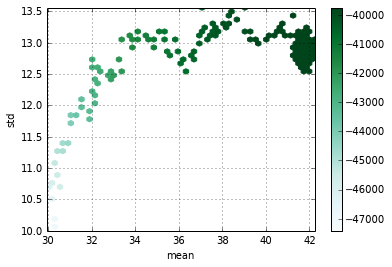The summary statistics are for post burn-in values in the chain only and are calculated off of the dark green region in the above plot. The plot above shows how accepted values of the mean and standard deviation move around the parameter space. Lighter shaded areas are low probability and notice the chain "converges" to the dark green area after exploring some higher age and std values. Note: other combinations of parameters were considered but weren't accepted in the chain.

The main point to consider is that the Bayesian Estimator is not merely the mean and standard deviation of age, but rather the estimated distribution as can be seen from the traceplots:

In :
#plot chains, excluding burn-in
time= np.arange(chain_length-burn_in)

# plot point estimate and confidence intervals:
plt.figure(figsize=(14, 10), dpi=200)
lw = 1

plt.subplot(221)
plt.ylabel("mu")
plt.plot(time, chain_ex['mean'].loc[burn_in:] , lw=lw)
plt.subplot(222)
chain_ex['mean'].loc[burn_in:].plot(kind='hist',bins=50,orientation='horizontal')

plt.subplot(223)
plt.ylabel("sigma")
plt.plot(time, chain_ex['std'].loc[burn_in:], lw=lw)
plt.subplot(224)
chain_ex['std'].loc[burn_in:].plot(kind='hist',bins=50,orientation='horizontal')

Out:
<matplotlib.axes.AxesSubplot at 0x6be0410>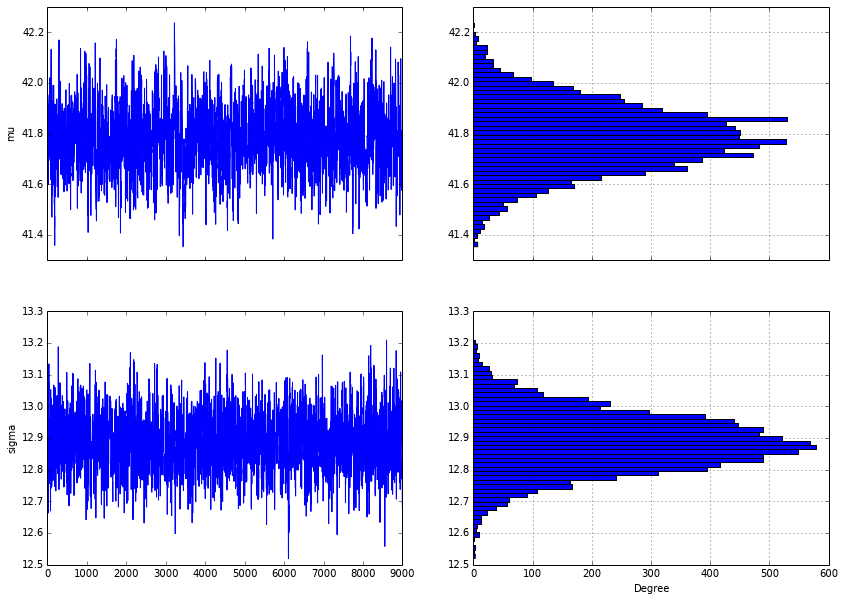For Bayesian Econometrics, convergence describes whether the chain oscillates randomly around a stable value (which is the mean of the distribution).

## Bayesian OLS Regression applied to Tobias and Koop

In :
# to make likelihood calculations easier, add a column of ones for the intercept:
tobias_koop['ones'] = 1


The Bayesian approach centers on probabilities of the posterior. We can recycle what we know from maximum likelihood to write the probability for individual i as

$Prob(\mathbf{b}| \mathbf{Y}_i, \mathbf{X}_i) = \frac{1}{\sqrt{2\sigma^2\pi}} e^{-\frac{(Y_i - \mathbf{X_i b})^2}{2\sigma^2}}$ which is just the normal pdf having mean $\mathbf{X}_i \mathbf{b}$

With that, we can write the log-likelihood function as $LogLike(\mathbf{b}| \mathbf{Y}, \mathbf{X}) = \sum_{i \in N} ln\left(Prob(\mathbf{b}| \mathbf{Y}_i, \mathbf{X}_i)\right)$ which is exactly the same as the log-likelihood, we'd use if we were estimating OLS by maximum likelihood. The following operationalizes this in code. Note that as in MLE, we have to estimate the $K$ parameters of $\mathbf{b}$ and our estimate for $\sigma$.

In :
# Likelihood Function for OLS (Bayesians call this log of posterior): identical to likelihood function for MLE
def log_likelihood(theta, x, y):
sigma = np.asmatrix(theta[-1])         #the last element of theta is the std dev of epsilon, and can't be <0
beta = np.asarray(theta[:-1])          #the first k are beta parameters
Y=np.asmatrix(tobias_koop[y])
if sigma <= 0:
return -np.inf                     #account for a chain trying to assign sigma < 0: penalize heavily
else:
mu = np.asmatrix(np.sum(tobias_koop[x]*np.tile(beta,(tobias_koop.shape,1)),axis=1)) #this is xb
mu =  mu.reshape(Y.shape,1)
prob = scipy.norm.pdf(Y,mu,sigma)
return sum(np.log(prob))


Now that we have defined the likelihood function (good for any OLS style model), we need to use an algorithm to sample parameters from the posterior. The MH algorithm has some advantages, but there are better ones out there that have been recently developed. For this example, we will use the emcee algorithm (http://dan.iel.fm/emcee/current/) that has been used in the Astronomy/Physics Literature. It wanders the parameter space using a more informed method rather than just a random walk (what the MH algorithm we employed uses). This code constructs a chain for our model parameters:

In :
x = ['educ','pexp','pexp2','broken_home','ones']
y = ['ln_wage']

# this calls the emcee mcmc algorithm
ndim, nwalkers = shape(x)+1, 100      #number of parameters, #of walkers in ensemble
nsteps, nburn = 5000, 1000              #total number of steps, # of burn-in steps

starting_guesses = np.random.rand(nwalkers, ndim)*.1

sampler = emcee.EnsembleSampler(nwalkers, ndim, log_likelihood, args=[x, y],threads=5)

store_chain=sampler.run_mcmc(starting_guesses, nsteps)


The estimator returns a Markov Chain (actually the Ensemble Sampler returns 100 chains (number of walkers)). Note: in what follows below, it should be evident that the Bayesian Approach estimates a distribution of parameter estimates rather than focusing on parameter estimates. As an aside, this calculates the likelihood function 5,000 x 100 = 500k times. This may be a little overkill for an OLS model.....

The following graphs one of these chains. Note: this exhibits periodicity which is problematic, so would need to look at combining across all chains to judge convergence. See discussion here: http://dan.iel.fm/emcee/current/user/line/

In :
#Plot the entire chain:
time= np.arange(nsteps-nburn)  # x values for plot (integer count)
sample = sampler.chain[:, nburn:, :]

# plot point estimate and confidence intervals:
plt.figure(figsize=(14, 10), dpi=200)
lw = 1

# to show the full chain, need to loop through walkers, but we'll just show the first walker:
plt.subplot(521)
plt.ylabel("Intercept")
plt.plot(time, sample[1,:,4], label="Intercept",lw=lw)
plt.subplot(522)
plt.hist(sample[1,:,4],lw=lw, label="Intercept", orientation='horizontal')

plt.subplot(523)
plt.ylabel("b_educ")
plt.plot(time, sample[1,:,0], label="b_educ",lw=lw)
plt.subplot(524)
plt.hist(sample[1,:,0],lw=lw, label="b_educ",orientation='horizontal')

plt.subplot(525)
plt.ylabel("b_pexp")
plt.plot(time, sample[1,:,1], label="b_pexp",lw=lw)
plt.subplot(526)
plt.hist(sample[1,:,1],lw=lw, label="b_pexp",orientation='horizontal')

plt.subplot(527)
plt.ylabel("b_pexp2")
plt.plot(time, sample[1,:,2], label="b_pexp2",lw=lw)
plt.subplot(528)
plt.hist(sample[1,:,2],lw=lw,label="b_pexp2", orientation='horizontal')

plt.subplot(529)
plt.ylabel("b_broken_home")
plt.plot(time,sample[1,:,3], label="b_broken_home",lw=lw)
plt.subplot(520)
plt.hist(sample[1,:,3],lw=lw, label="b_broken_home",orientation='horizontal')

Out:
(array([    5.,    55.,   231.,   709.,  1072.,   937.,   649.,   270.,
49.,    23.]),
array([-0.13442667, -0.10919383, -0.083961  , -0.05872817, -0.03349534,
-0.0082625 ,  0.01697033,  0.04220316,  0.06743599,  0.09266882,
0.11790166]),
<a list of 10 Patch objects>)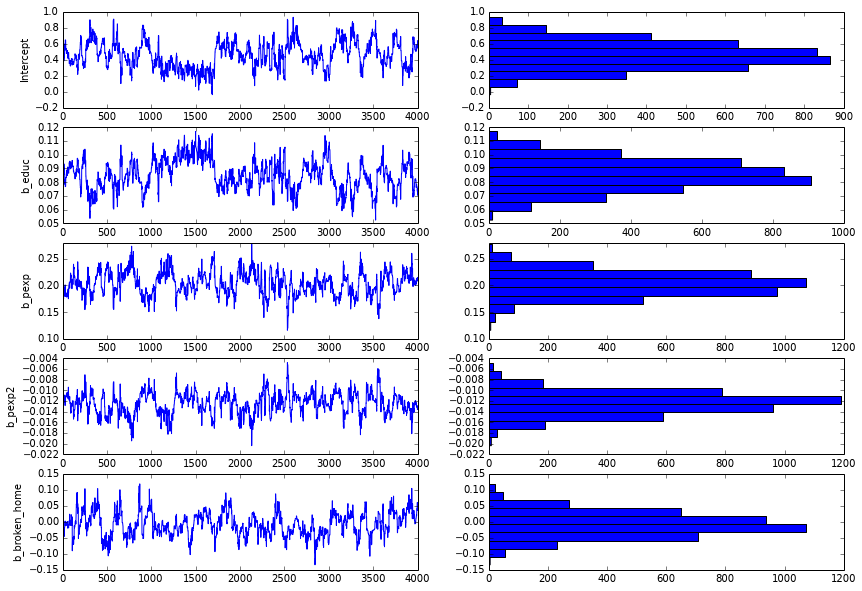While the trace plots above look fairly similar to what we found above in the bootstrapping excercise, we came at this information from a very different perspective, by sampling values from the posterior distribution. This was done by evaluating a number of trial values of our parameters and settling on areas of the parameter space having higher probability values for the posterior.

What is of more interest is the information contained in all of the chains, and what it tells us about the distribution of the parameters. The following trace chart uses all of the information from all 100 chains.

In :
# to show the full chain, need to loop through walkers
walkers=np.arange(nwalkers)

plt.figure(figsize=(14, 14), dpi=200)

lw = .05

for i in walkers:
plt.subplot(511)
plt.ylabel("Intercept")
plt.plot(time, sample[i,:,4], label="Intercept",lw=lw)

plt.subplot(512)
plt.ylabel("b_educ")
plt.plot(time, sample[i,:,0], label="b_educ",lw=lw)

plt.subplot(513)
plt.ylabel("b_pexp")
plt.plot(time, sample[i,:,1], label="b_pexp",lw=lw)

plt.subplot(514)
plt.ylabel("b_pexp2")
plt.plot(time, sample[i,:,2], label="b_pexp2",lw=lw)

plt.subplot(515)
plt.ylabel("b_broken_home")
plt.plot(time,sample[i,:,3], label="b_broken_home",lw=lw)